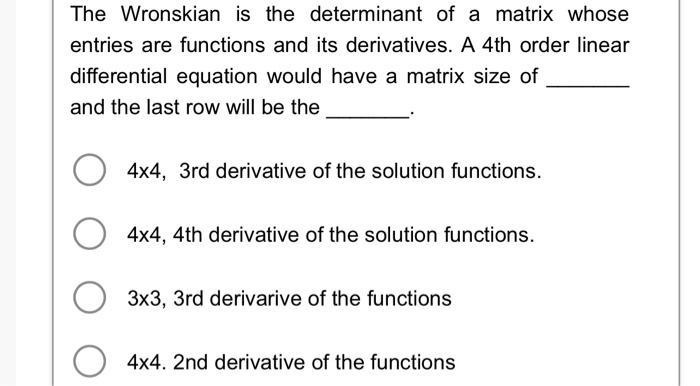# Question The Wronskian is the determinant of a matrix whose entries are functions and its derivatives. A 4th order linear differential equation would have a matrix size of and the last row will be the $$4 \times 4,3$$ rd derivative of the solution functions. $$4 \times 4,4$$ th derivative of the solution functions. $$3 \times 3$$, 3rd derivarive of the functions 4x4. 2 nd derivative of the functionsTranscribed Image Text: The Wronskian is the determinant of a matrix whose entries are functions and its derivatives. A 4th order linear differential equation would have a matrix size of and the last row will be the $$4 \times 4,3$$ rd derivative of the solution functions. $$4 \times 4,4$$ th derivative of the solution functions. $$3 \times 3$$, 3rd derivarive of the functions 4x4. 2 nd derivative of the functions
Transcribed Image Text: The Wronskian is the determinant of a matrix whose entries are functions and its derivatives. A 4th order linear differential equation would have a matrix size of and the last row will be the $$4 \times 4,3$$ rd derivative of the solution functions. $$4 \times 4,4$$ th derivative of the solution functions. $$3 \times 3$$, 3rd derivarive of the functions 4x4. 2 nd derivative of the functions&#12304;General guidance&#12305;The answer provided below has been developed in a clear step by step manner.Step1/1ExplanationThe wronskian is the determinant of a matrix whose entries are function and its derivative. A 4th order linear differential equation is $$\mathrm{\frac{{{d}^{{4}}{y}}}{{\left.{d}{x}\right.}^{{4}}}+{P}{\left({x}\right)}\frac{{{d}^{{3}}{y}}}{{\left.{d}{x}\right.}^{{3}}}+{Q}{\left({x}\right)}\frac{{{d}^{{2}}{y}}}{{\left.{d}{x}\right.}^{{2}}}+{R}{\left({x}\right)}\frac{{{\left.{d}{y}\right.}}}{{\left.{d}{x}\right.}}+{S}{\left({x}\right)}{y}={0}}$$Then, the linear differential equation have four linearly independent solution as y_{1}(x) ,y_{2}(x), y_{3}(x) and y_{4}(x).Now the wronskian is \( \begin{align*} \mathrm{{W}{\left({y}_{{{1}}},{y}_{{{2}}},{y}_{{{3}}},{y}_{{{4}}}\right)}{\left({x}\right)}} &= \mathrm{{\left[\begin{matrix}{y}_{{{1}}}{\left({x}\right)}&{y}_{{{2}}}{\left({x}\right)}&{y}_{{{3}}}{\left({x}\right)}&{y}_{{{4}}}{\left({x}\right)}\\\frac{{{\left ... See the full answer